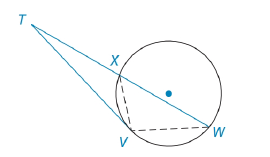Chapter 6.3, Problem 49EElementary Geometry For College St...

7th Edition
Alexander + 2 others
ISBN: 9781337614085

Solutions

Chapter
SectionElementary Geometry For College St...

7th Edition
Alexander + 2 others
ISBN: 9781337614085
Textbook Problem

In Exercises 46 to 49, prove the stated theorem.If a tangent segment and a secant segment are drawn to a circle from an external point, then the square of the length of the tangent equals the product of the length of the secant with the length of its external segment. See Figure 6.52 on page 303.To determine

To find:

To prove the following stated theorem,

“If a tangent segment and a secant segment are drawn to a circle from an external point, then the square of the length of the tangent equals the product of the length of the secant with the length of its external segment”.

Explanation

Given that tangent EA¯ with point of tangency A and secant ED¯.

To prove (EA)2=EDEC(PS)2=PRPQ

The diagrammatic representation is given below,

Then, ADE is an inscribed angle, therefore, mADE=12mAC^

Still sussing out bartleby?

Check out a sample textbook solution.

See a sample solution

The Solution to Your Study Problems

Bartleby provides explanations to thousands of textbook problems written by our experts, many with advanced degrees!

Get Started

x3a+b3

Applied Calculus for the Managerial, Life, and Social Sciences: A Brief Approach